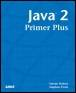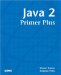# Java Class LibrariesJava™ 2 Primer PlusBy Steven Haines, Steve Potts Table of Contents Chapter 5.  Methods

This chapter began discussion about the idea of divide and conquer, and how you can create reusable methods that encapsulate the functionality you might want to use in the future. From this concept you are going to develop a set of methods over the next few years that other people are probably developing as well. Because everyone is going to need a square method, would it be nice if someone created this method and published it for everyone to use?

After you understand the basic syntax and semantics of the Java programming language, a big part of the learning curve is locating all the prebuilt classes and methods that you have been created for you. Java has implemented most of the basic functionality that you could ever think of developing on your own. The Java 2 SDK contains classes that will do much of your work for you!

The best resource you can have as a Java programmer is a link to Java's Documentation, referred to as Javadoc. Javadoc is a set of HTML-based documentation for the Java 2 SDK and through a mechanism you will learn about later, you can document your own classes and methods to share with others (and to understand six months from now when you have completely forgotten what a method does).

The Javadoc for the Java 2 SDK can be found at http://java.sun.com/docs/index.html.

Find the version of the SDK you are using and follow the links to see the documentation for your version. You can also download a copy of the documentation to install on you own computer, which is highly recommended if you have the space.

There are hundreds of classes you can look at, but because of the heavy focus on mathematical operations in this chapter, this section will present Java's Math class. The Math class defines two very important fields: E and PI, so if you are computing natural logarithms or need to use PI you can access them by referencing the explicit name of the Math class, appending a period to it, and then referencing the field you are interested in, as follows:

` int radius = 5;  float area = Math.PI * square( radius ); `

The Math class also defines a set of methods, as shown in Table 5.2.

##### Table 5.2. Math Methods

Method

Description

double abs(double a)

Returns the absolute value of a double value.

float abs(float a)

Returns the absolute value of a float value.

int abs(int a)

Returns the absolute value of an int value.

long abs(long a)

Returns the absolute value of a long value.

double acos(double a)

Returns the arc cosine of an angle, in the range of 0.0 through pi.

double asin(double a)

Returns the arc sine of an angle, in the range of pi/2 through pi/2.

double atan(double a)

Returns the arc tangent of an angle, in the range of pi/2 through pi/2.

double atan2(double a, double b)

Converts rectangular coordinates (b, a) to polar (r, theta).

double ceil(double a)

Returns the smallest (closest to negative infinity) double value that is not less than the argument and is equal to a mathematical integer.

double cos(double a)

Returns the trigonometric cosine of an angle.

double exp(double a)

Returns the exponential number e (that is, 2.718…) raised to the power of a double value.

double floor(double a)

Returns the largest (closest to positive infinity) double value that is not greater than the argument and is equal to a mathematical integer.

double IEEEremainder(double f1, double f2)

Computes the remainder operation on two arguments as prescribed by the IEEE 754 standard.

double log(double a)

Returns the natural logarithm (base e) of a double value.

double max(double a, double b)

Returns the greater of two double values.

float max(float a, float b)

Returns the greater of two float values.

int max(int a, int b)

Returns the greater of two int values.

long max(long a, long b)

Returns the greater of two long values.

double min(double a, double b)

Returns the smaller of two double values.

float min(float a, float b)

Returns the smaller of two float values.

int min(int a, int b)

Returns the smaller of two int values.

long min(long a, long b)

Returns the smaller of two long values.

double pow(double a, double b)

Returns of value of the first argument raised to the power of the second argument.

double random()

Returns a double value with a positive sign, greater than or equal to 0.0 and less than 1.0.

double rint(double a)

Returns the double value that is closest in value to a and is equal to a mathematical integer.

long round(double a)

Returns the closest long to the argument.

int round(float a)

Returns the closest int to the argument.

double sin(double a)

Returns the trigonometric sine of an angle.

double sqrt(double a)

Returns the correctly rounded positive square root of a double value.

double tan(double a)

Returns the trigonometric tangent of an angle.

double toDegrees(double angrad)

Converts an angle measured in radians to the equivalent angle measured in degrees.

double toRadians(double angdeg)

Converts an angle measured in degrees to the equivalent angle measured in radians.

All these methods are static, so you do not need to create an instance of the Math class to use them, simply reference them as Math-period-method_name, for example:

` double result = Math.tan( angle ); `

Spend some time looking through these methods, experiment, and see what you can come up with.

 TopJava 2 Primer Plus
ISBN: 0672324156
EAN: 2147483647
Year: 2001
Pages: 332

Similar book on Amazon

flylib.com © 2008-2017.
If you may any questions please contact us: flylib@qtcs.net